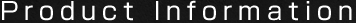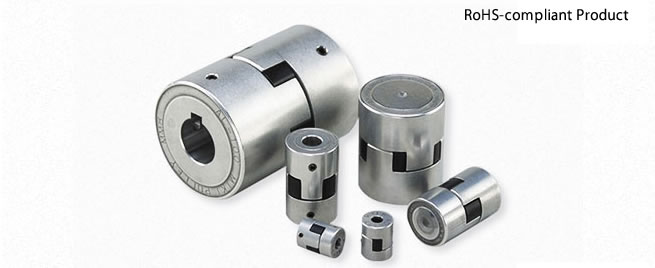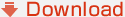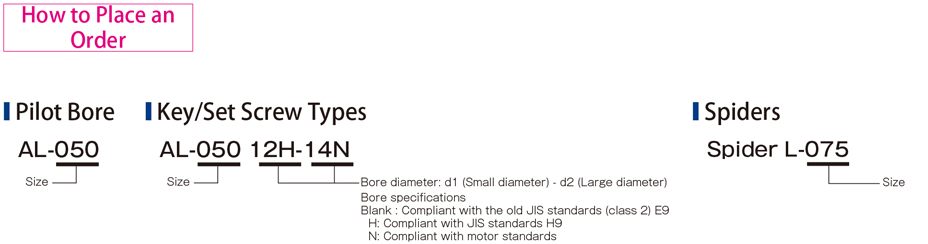﻿ Specifications | AL Models | Miki Pulley# AL Models## AL Models Specifications

AL Models

[Specifications]

ModelTorqueMisalignmentMax. rotation speed ［min-1Moment of inertia ［kg・m2Mass ［kg］
Nominal ［N・m］Max. ［N・m］Parallel ［mm］Angular ［°］Axial ［mm］
AL-0350.51.50.10.5＋0.3180000.38×10-60.01
AL-0501.54.50.21.0±0.5120005.10×10-60.06
AL-070390.21.0±0.590001.79×10-50.12
AL-0755150.21.0±0.570005.36×10-50.21
AL-0908240.31.0±0.560001.15×10-40.31
AL-09510300.31.0±0.560001.40×10-40.36
AL-10025750.31.0±0.750004.34×10-40.78
AL-110501500.31.0±0.740001.43×10-31.56

*Max. rotation speed does not take into account dynamic balance or mounting misalignment.

*The moment of inertia and mass are measured for the pilot bore.

[Dimensions]

couplingsUnit ［mm］
Modeld1, d2DLL1L2
Pilot boreMin.Max.
AL-03544816.120.56.57.5*1
AL-05056162743.215.512.2
AL-07056203549.218.512.2
AL-07557264554.421.012.4
AL-09059285455.021.013.0
AL-09559285561.024.013.0
AL-100511366688.035.018.0
AL-1105114885110.044.022.0

*"Pilot bore" refers to center processing. Minimums and maximums for d1 and d2 are values at the MIKI PULLEY standard hole-drilling standards.

*The value marked *1 leaves a 1 mm space for the thickness of the spider body.

[Dimensions]

SpiderUnit ［mm］
Coupling ModelSpider ModelL2RK
AL-035L-0356.5
AL-050L-05012.2
AL-070L-07012.2
AL-075L-07512.4206.0
AL-090L-090/09513.0226.3
AL-095L-090/09513.0226.3
AL-100L-10018.0266.0
AL-110L-11022.0306.0

[Standard Hole-drilling Standards]

Unit ［mm］
Models compliant with the old JIS standards (Class 2)Models compliant with the new JIS standardsModels compliant with the new motor standards
Nominal bore diameterBore diameter (d1, d2)Keyway width (W1, W2)Keyway height (T1, T2)Set screw hole (M)Nominal bore diameterBore diameter (d1, d2)Keyway width (W1, W2)Keyway height (T1, T2)Set screw hole (M)Nominal bore diameterBore diameter (d1, d2)Keyway width (W1, W2)Keyway height (T1, T2)Set screw hole (M)
ToleranceH7, H8E9+0.30-ToleranceH7H9+0.30-ToleranceG7, F7H9+0.30-
66+0.0180--2-M4----------
77+0.0220--2-M4----------
88+0.0220--2-M4----------
99+0.0220--2-M4----------
1010+0.0220--2-M4----------
1111+0.0180--2-M4----------
1212+0.01804+0.050+0.02013.52-M412H12+0.01804+0.030013.82-M4-----
1414+0.01805+0.050+0.02016.02-M414H14+0.01805+0.030016.32-M414N14+0.024+0.0065+0.030016.32-M4
1515+0.01805+0.050+0.02017.02-M415H15+0.01805+0.030017.32-M4-----
1616+0.01805+0.050+0.02018.02-M416H16+0.01805+0.030018.32-M4-----
1717+0.01805+0.050+0.02019.02-M417H17+0.01805+0.030019.32-M4-----
1818+0.01805+0.050+0.02020.02-M418H18+0.01806+0.030020.82-M5-----
1919+0.02105+0.050+0.02021.02-M419H19+0.02106+0.030021.82-M519N19+0.028+0.0076+0.030021.82-M5
2020+0.02105+0.050+0.02022.02-M420H20+0.02106+0.030022.82-M5-----
2222+0.02107+0.061+0.02525.02-M622H22+0.02106+0.030024.82-M5-----
2424+0.02107+0.061+0.02527.02-M624H24+0.02108+0.036027.32-M624N24+0.028+0.0078+0.036027.32-M6
2525+0.02107+0.061+0.02528.02-M625H25+0.02108+0.036028.32-M6-----
2828+0.02107+0.061+0.02531.02-M628H28+0.02108+0.036031.32-M628N28+0.028+0.0078+0.036031.32-M6
3030+0.02107+0.061+0.02533.02-M630H30+0.02108+0.036033.32-M6-----
3232+0.025010+0.061+0.02535.52-M832H32+0.025010+0.036035.32-M8-----
3535+0.025010+0.061+0.02538.52-M835H35+0.025010+0.036038.32-M8-----
3838+0.025010+0.061+0.02541.52-M838H38+0.025010+0.036041.32-M838N38+0.050+0.02510+0.036041.32-M8
4040+0.025010+0.061+0.02543.52-M840H40+0.025012+0.043043.32-M8-----
4242+0.025012+0.075+0.03245.52-M842H42+0.025012+0.043045.32-M842N42+0.050+0.02512+0.043045.32-M8
4545+0.025012+0.075+0.03248.52-M845H45+0.025014+0.043048.82-M10-----
4848+0.025012+0.075+0.03251.52-M848H48+0.025014+0.043051.82-M1048N48+0.050+0.02514+0.043051.82-M10

*The ø11 or below requirement under the new JIS standards and ø11 requirement for the new motor standards are the same as the old JIS standards (class 2).

*For AL-035, the tolerance is +0.05+0 regardless of bore diameter. The set screw size is M3.

[Distance from set screw edge]

ModelAL-035AL-050AL-070AL-075AL-090AL-095AL-100AL-110
Distance from set screw edge C ［mm］3.57.591012121215﻿